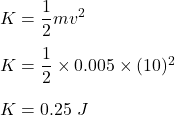## Si tomamos como referencia el recipiente uno y se aplican 5 gramos de tinta y el agua se moviera a una velocidad de 10m/s cual seria la ener

Question

Si tomamos como referencia el recipiente uno y se aplican 5 gramos de tinta y el agua se moviera a una velocidad de 10m/s cual seria la energia cinetica de la solucion

in progress 0
6 months 2021-07-28T03:00:20+00:00 1 Answers 2 views 0

Kinetic energy = 0.25 J

Explanation:

The question is “If we take container one as a reference and apply 5 grams of ink and the water moves at a speed of 10m / s, what would the kinetic energy of the solution be?”

We have,

Mass of ink, m = 5 grams = 0.005 kg

Speed of water, v = 10 m/s

We need to find the kinetic energy of the solution of water and ink. Kinetic energy is associated with the motion of an object. Its formula is given by :So, the kinetic energy of the solution is 0.25 Joules.